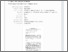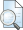## AKAR ke-m DARI MATRIKS KOMPLEKS NON SINGULAR

ARIFAH, NUR (2009) AKAR ke-m DARI MATRIKS KOMPLEKS NON SINGULAR. Other thesis, University of Muhammadiyah Malang.Preview
Text
AKAR_ke.pdf - Published Version

## Abstract

One of a problem in matrix is how to find the m-th roots of non-singular complex matrix. To solve the problem, there are some steps regording to eigen value and vector from a matrix. Asumed that polinomil matrix P( λ) = In λm – A, where In is matrix identity from order n and λ is a variabel complex. A matrix B ∈ Mn is the m—th roots from A if and only if P(B) =Bm-A=0. As a result, problem from the m-th roots from A is highly related with spectral analysis from ) ( λ Ρ If an n x n complex matrix A is non-singular, then for every integer m > 1, A has an m-th root B.i.e., Bm = A, we can construct m-th roots of A the form 1 ) ( − Α Ρ Α Υ Υ = Β λ J ,where YA is Jordan Chain of P( λ).

Item Type: Thesis (Other) L Education > L Education (General) Faculty of Teacher Training and Education > Department of Mathematics Education (84202) Anwar Jasin 21 Mar 2012 15:36 21 Mar 2012 15:36 http://eprints.umm.ac.id/id/eprint/887View Item